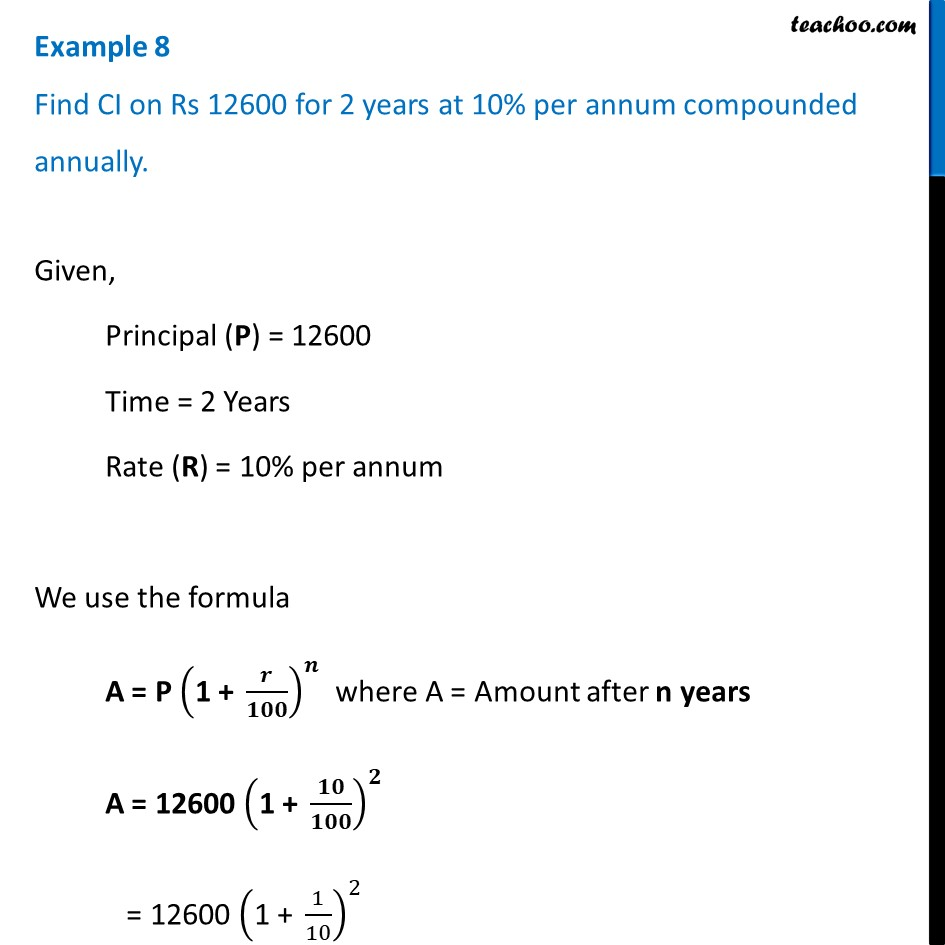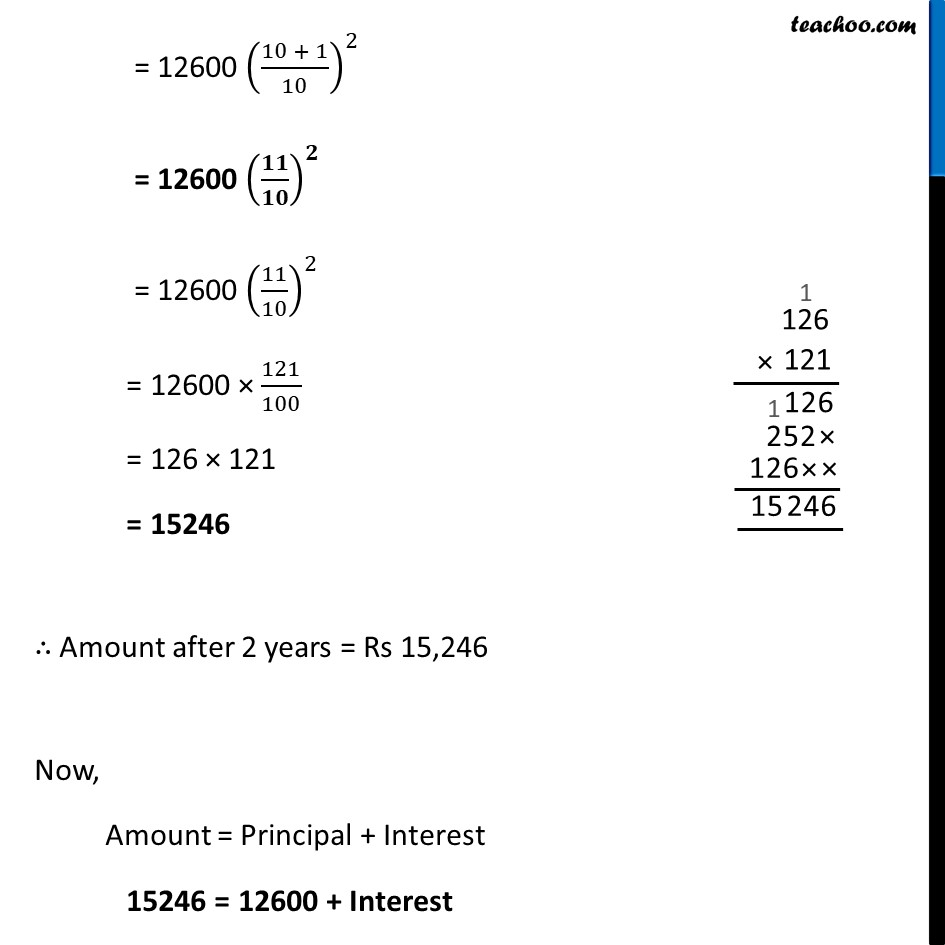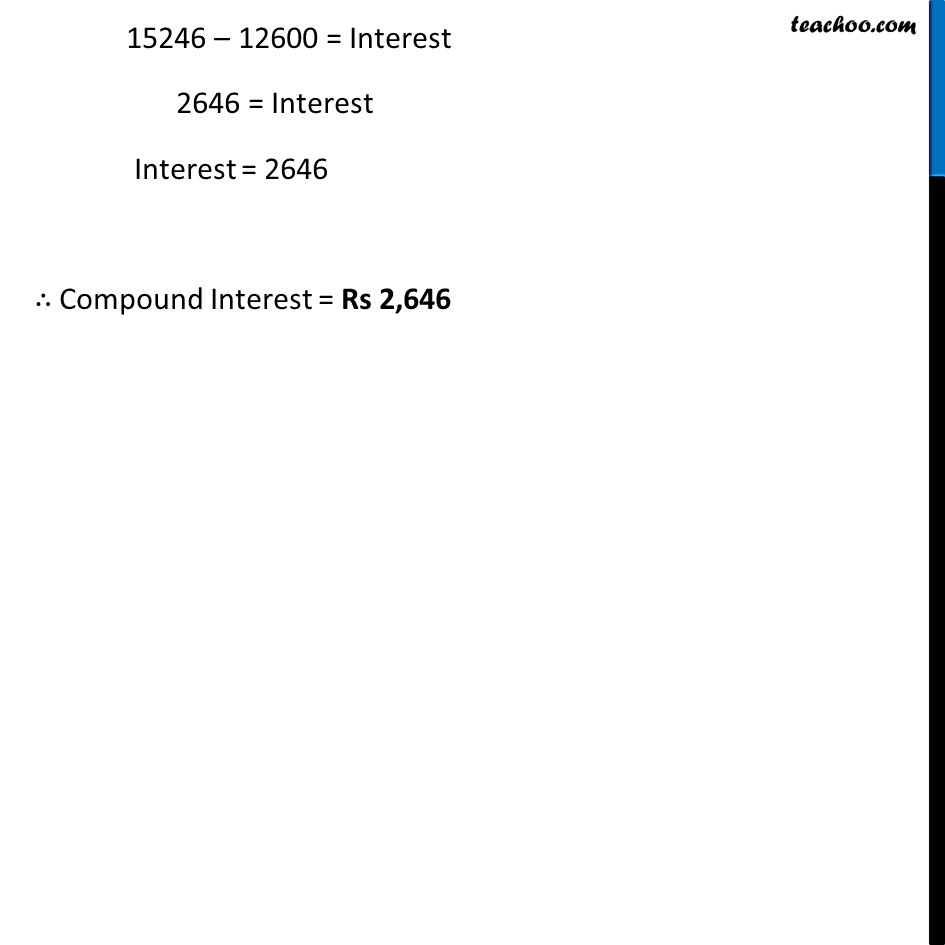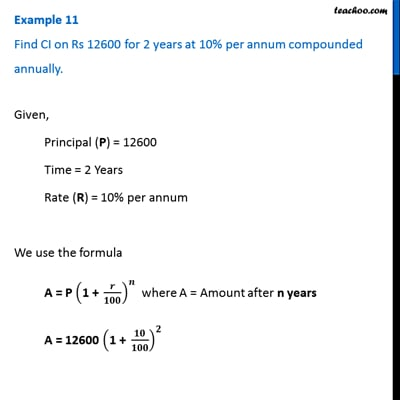Examples

Chapter 7 Class 8 Comparing Quantities
Serial order wiseThis video is only available for Teachoo black users

Learn in your speed, with individual attention - Teachoo Maths 1-on-1 Class

### Transcript

Example 8 Find CI on Rs 12600 for 2 years at 10% per annum compounded annually.Given, Principal (P) = 12600 Time = 2 Years Rate (R) = 10% per annum We use the formula A = P ("1 + " 𝒓/𝟏𝟎𝟎)^𝒏 where A = Amount after n years A = 12600 ("1 + " 𝟏𝟎/𝟏𝟎𝟎)^𝟐 = 12600 ("1 + " 1/10)^2 = 12600 ((10 + 1)/10)^2 = 12600 (𝟏𝟏/𝟏𝟎)^𝟐 = 12600 (11/10)^2 = 12600 × 121/100 = 126 × 121 = 15246 ∴ Amount after 2 years = Rs 15,246 Now, Amount = Principal + Interest 15246 = 12600 + Interest 15246 – 12600 = Interest 2646 = Interest Interest = 2646 ∴ Compound Interest = Rs 2,646